# 集群语言 Python 入门 脚本工具

### 文章目录

• 脚本工具 Python
• Python的特点
• Python是一个下限特别低, 上限特别高的编程语言
• Python支持多种编程风格
• Python语法简洁, 表达能力强
• 编程语言排行榜
• 解释运行
• 跨平台
• 可扩展强
• 可嵌入
• 丰富的库
• Python能做哪些事
• 对于开发人员
• 对于测试人员
• 对于运维人员
• 对于产品经理
• 对于黑客/白帽子
• 对于其他人
• 除了驱动开发, Python几乎无所不能.
• Python的缺点 Python一时爽，重构火葬场
• Python安装我想出视频哈哈水水
• 一行代码带你玩自恋
• Python快速入门
• 变量和赋值
• 动态类型
• 动态类型的体现
• 变量命名规则
• 认识 "数字"
• 认识 "字符串"
• 认识 "布尔类型"

•# 脚本工具 Python

优雅 明确 简单

## Python是一个下限特别低, 上限特别高的编程语言

1. 下限特别低: 上手容易, 特别适合啥都不懂的小白作为第一门编程语言. 通过简单的代码就能做出实用的功能, 特别容易培养兴趣.
2. 上限特别高: Python有非常丰富的语法特性, 支持多种编程风格, 又有非常庞大驳杂的库. 想要学到 “精通” 也绝非一朝一夕.

## Python支持多种编程风格

1. Python支持面向过程的编程风格. 如果你熟悉C语言, 那么完全可以像写C一样写Python 好的本人试试
2. Python支持面向对象的编程风格. 数字, 字符串, 函数, 模块… 都是 “对象”.
3. Python支持函数式编程. 其实这世界上只有两种编程语言,C类语言, Lisp类语言. Python也支持函数式编程.

## Python语法简洁, 表达能力强

python代码量小, 维护成本低, 编程效率高, 标准库强大, 第三方库非常丰富, 目前已经成为全世界最广泛使用的编程语言之一.

同一样问题，用不同的语言解决，代码量差距太多了，一般情况下python是java的1/5，所以说人数苦短，我用python，多留点时间泡妹子吧，不然就老了.

### 编程语言排行榜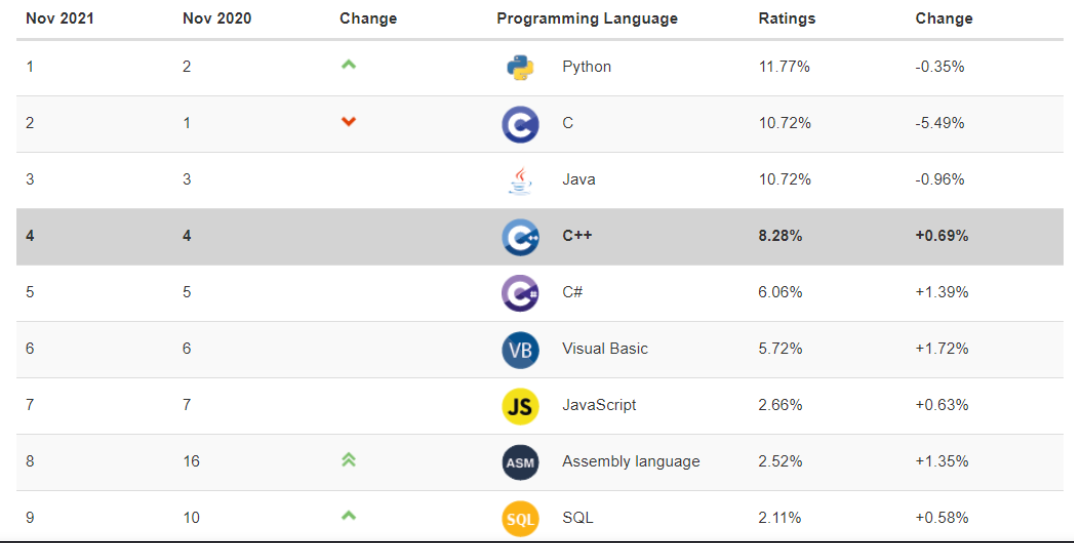我们可以非常直观的看到c与c++排第一的，甩python几条街，哈哈，因为我们应聘职位的时候简历上都是写c/c++工程师，没有分开过不是吗？大佬都是低调的，不过python也不赖，可以看出比java高了。python抓住了三大机遇，时代造就了它 1.web开发 2.科学计算领域 3.人工智能当然也错过了绝杀java的机会，那就是移动开发，反正89年划时代的一年，很牛逼

### 解释运行

Python是一种解释型的编程语言. 和C/C++不同, 不是先将源代码文件转化成可执行文件, 再执行; 而是直接由Python解释器一行一行的读取源代码, 每读一行就执行一行.
但严格意义上讲, Python算是一种 “半编译, 半解释” 型的语言. 一方面, Python解释器会按行读取源代码文件, 然后会先将源代码转为供Python解释器直接执行的 “字节码”. 然后再执行字节码.

例如, 我们执行一个.py文件之后, 会生成一个同名的.pyc文件. 这个.pyc文件就是Python解释器生成的字节码文件. 如果已经存在了.pyc文件, 那么就不需要再 “翻译” 一次了, 这样也提高了执行效率.

### 跨平台

Python是基于Python的解释器来进行执行. 只要某个操作系统/平台上能运行Python解释器, 就可以完美的运行Python的源代码. 主流的Windows, Linux, Mac等操作系统上都能够很好的支持Python.

### 可扩展强

Python可以很容易的调用C/C++语言. 如果觉得哪里的逻辑性能不满足要求, 可以用C/C++重构这部分模块, 用Python调用.

### 可嵌入

Python的代码也可以很容易的嵌入到C/C++中执行.

### 丰富的库

君子性非异也, 善假于物也.
Python标准库已经非常丰富强大, 同时也有非常庞大的第三方库. 几乎可以是上天入地, 心随我意~

## Python能做哪些事

### 对于开发人员

1. 模块组合拼接, 快速搭建线上业务逻辑
2. 实现线下数据生成流程
3. 爬虫开发
4. web开发
5. 机器学习
6. 大数据处理

### 对于测试人员

1. 搭建自动化测试框架
2. 搭建持续集成环境
3. 搭建bug状态跟踪平台

1. 自动化机器管理
2. 自动化上线部署
3. 数据同步分发工具

### 对于产品经理

1. 数据统计/分析
2. office辅助工具

1. 渗透测试工具
2. 系统分析工具
3. 实现游戏外挂

### 对于其他人

1. 实现各种解决我们生活中的具体问题的小工具

## Python的缺点 Python一时爽，重构火葬场

执行效率!! 执行效率!! 执行效率!!

1. 在摩尔定律的作用下, 硬件越来越便宜, 反而是开发人员的成本越来越高;
2. 一些好的编程实践可以帮助我们写出比较高性能的代码;
3. python解释器的执行效率也在不断被优化;

## Python安装我想出视频哈哈水水

记住2，3是分水岭

哈哈 hello world最简单写法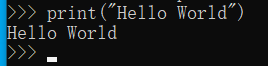### 一行代码带你玩自恋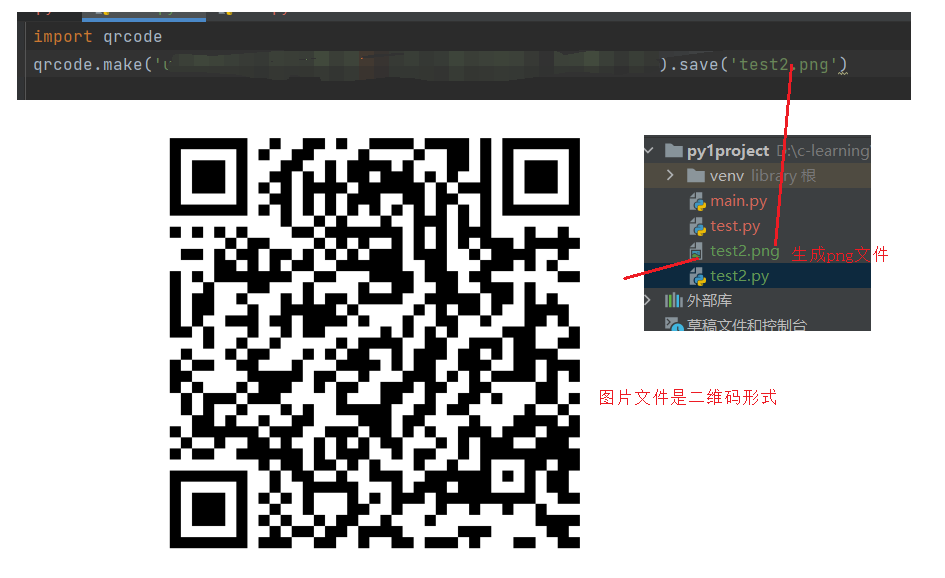自己扫扫看哈，挺好玩的哈哈

# Python快速入门

## 变量和赋值

### 动态类型

1. Python中的变量不需要声明, 直接定义即可. 会在初始化的时候决定变量的 “类型” 到了这里我就想到一个好玩的东西，你的身体大小决定你的影子的大小，还是影子的大小决定你身体的大小 这里的python给我的感觉就是影子决定身体的大小
2. 使用 = 来进行初始化和赋值操作.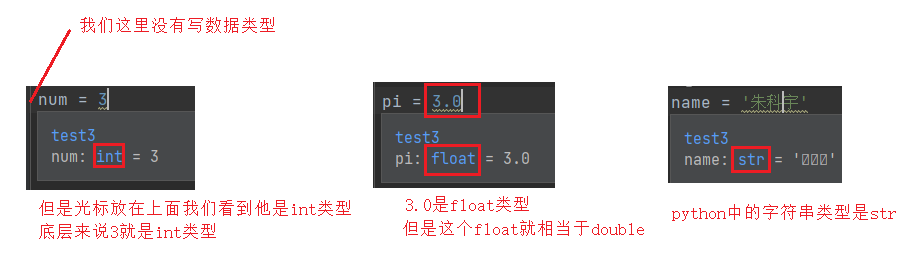``````num = 3
pi = 3.0
name = '朱科宇'
``````

Python中也支持增量赋值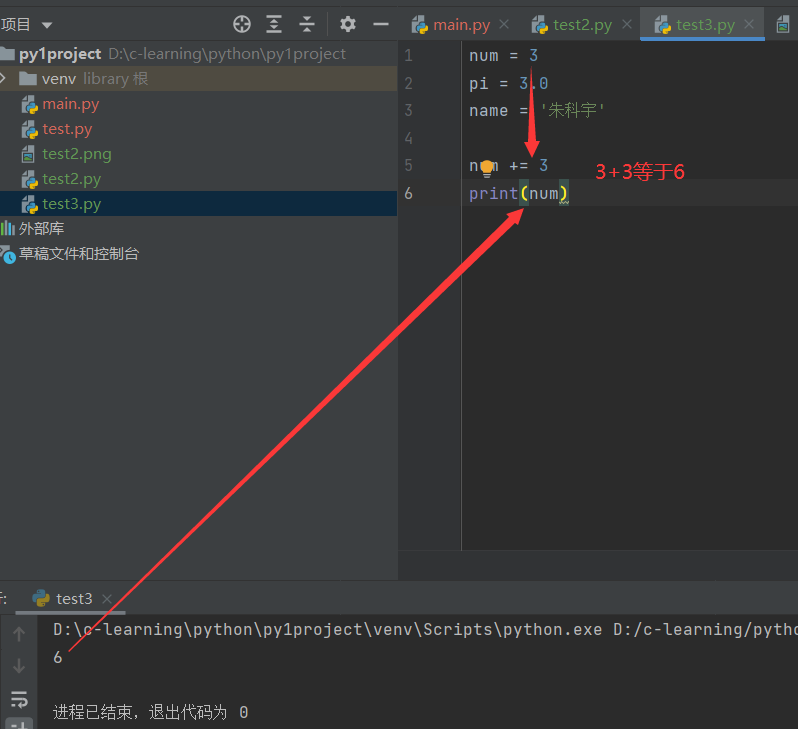``````num = 3
num += 3
print(num)
``````

Python中不支持 ==++/–==这样的操作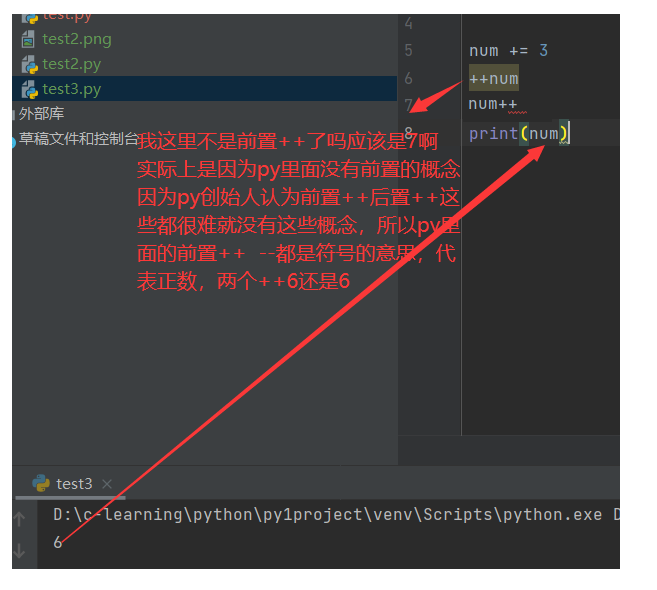#### 动态类型的体现

一个变量在运行的过程中，类型可以发生变化

同一个名字变量, 可以赋值成不同的类型的值.这也就是变量前面不写变量类型的原因，因为他是动态的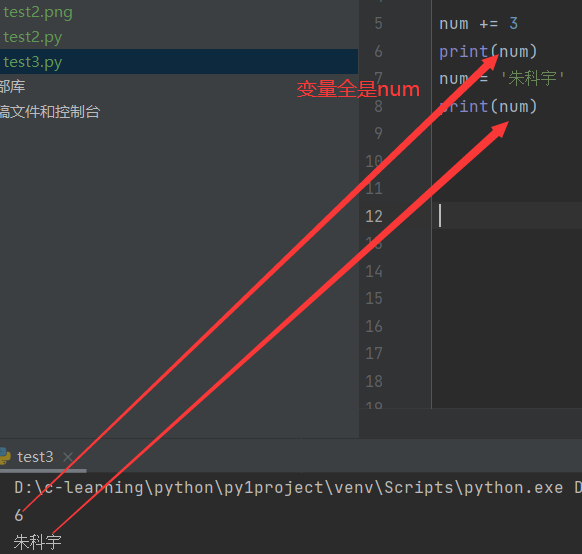``````num = 3
num += 3
print(num)
num = '朱科宇'
print(num)
``````

### 变量命名规则

1. 变量名必须是字母, 数字, 下划线. 但是不能用数字开头(规则和C语言一样).
2. 变量名大小写敏感, case 和 Case 是两个不同的变量.
3. 变量命名要做到 “见名知意” .

## 认识 “数字”

Python没有int, float, 这样的关键字, 但是实际上数字的类型是区分 “int” “float” 这样的类型的. 使用内建函数 type (py自带的函数，不要其他的模块就能直接使用)可以查看变量的类型.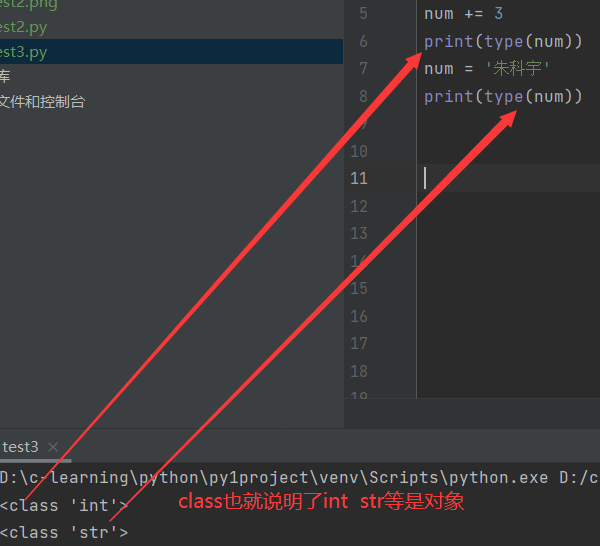``````num += 3
print(type(num))
num = '朱科宇'
print(type(num))
``````

Python中的数字变量的取值范围, 并没有限制(完全取决于你机器的内存多大), 而不是像C语言中int用4个字节表示.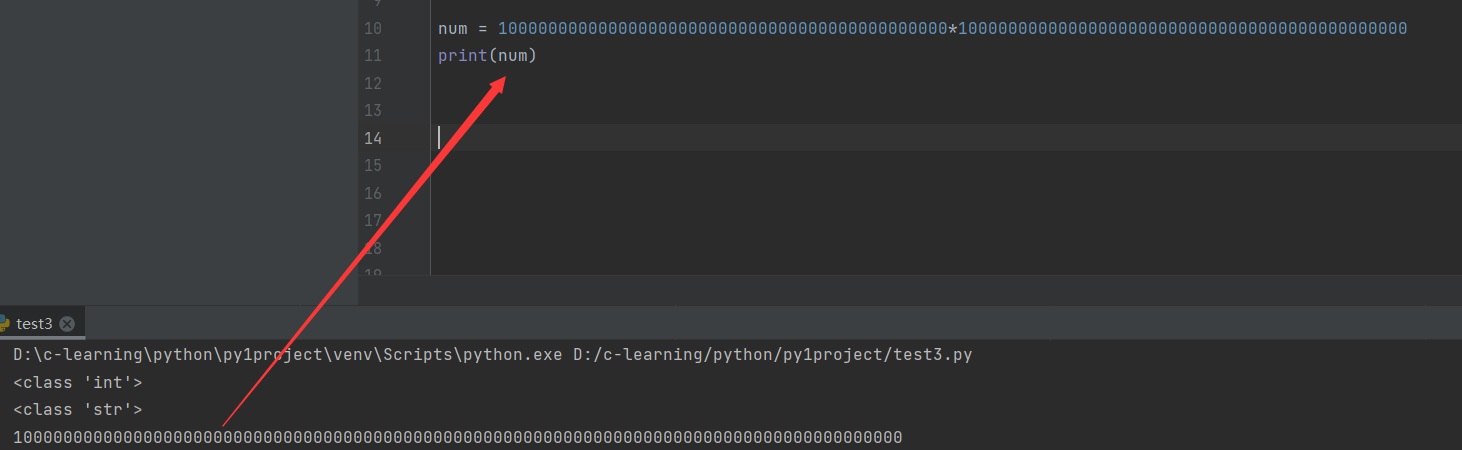``````num = 100000000000000000000000000000000000000000000*100000000000000000000000000000000000000000000
print(num)
``````

## 认识 “字符串”

Python中可以使用 单引号(’), 双引号("), 三引号(’’’/""") 来表示字符串. 这三种字符串的区别, 我们后面再讨论.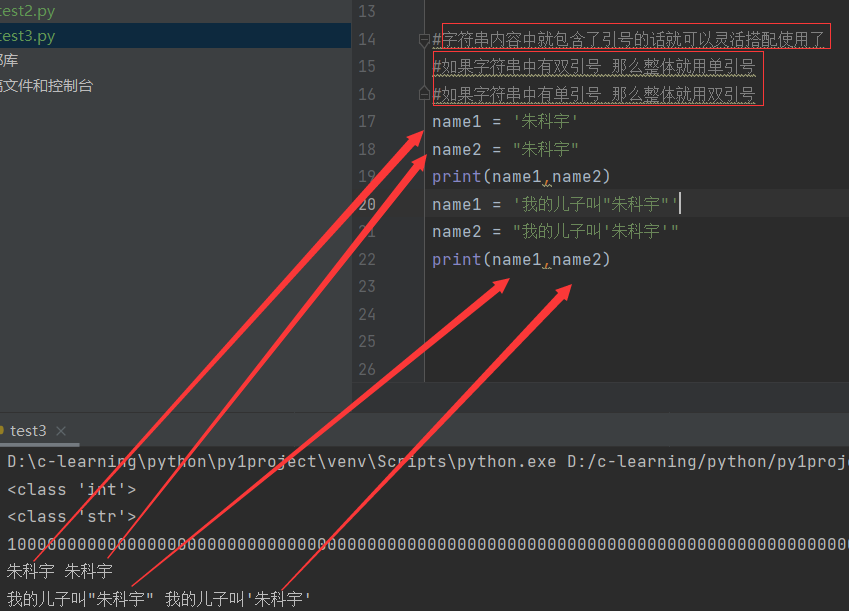``````#字符串内容中就包含了引号的话就可以灵活搭配使用了
#如果字符串中有双引号 那么整体就用单引号
#如果字符串中有单引号 那么整体就用双引号
name1 = '朱科宇'
name2 = "朱科宇"
print(name1,name2)
name1 = '我的儿子叫"朱科宇"'
name2 = "我的儿子叫'朱科宇'"
print(name1,name2)
``````

那么字符串中同时有单引号和双引号呢，我们还可以用三引号括起来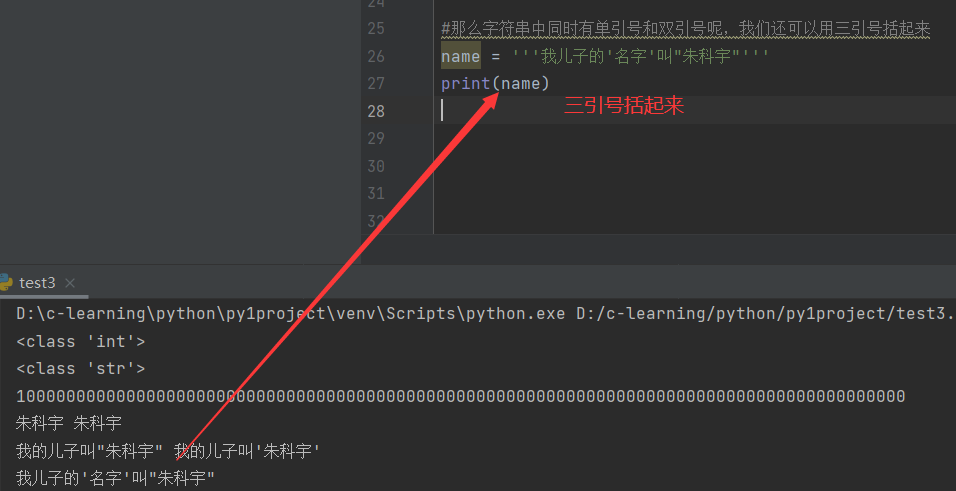``````#那么字符串中同时有单引号和双引号呢，我们还可以用三引号括起来
name = '''我儿子的'名字'叫"朱科宇"'''
print(name)
``````

内建函数 len 获取字符串长度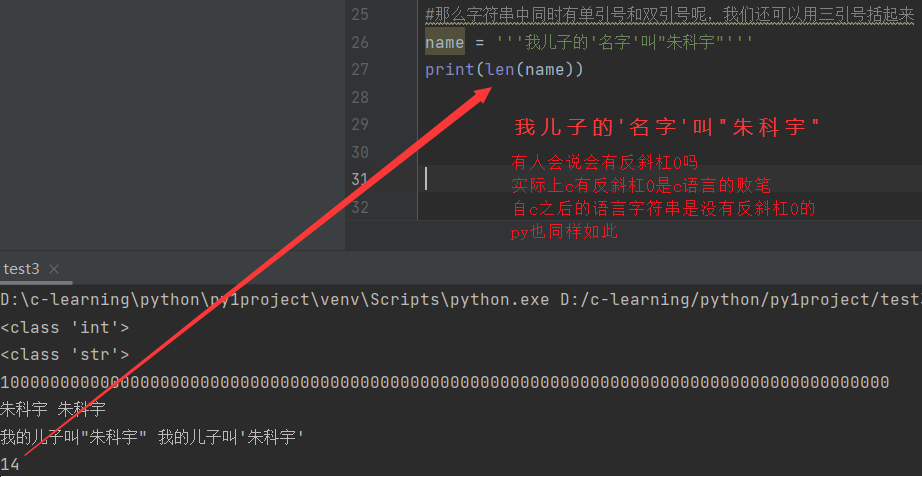``````#那么字符串中同时有单引号和双引号呢，我们还可以用三引号括起来
name = '''我儿子的'名字'叫"朱科宇"'''
print(len(name))
``````

索引操作符[] 找下标对应的数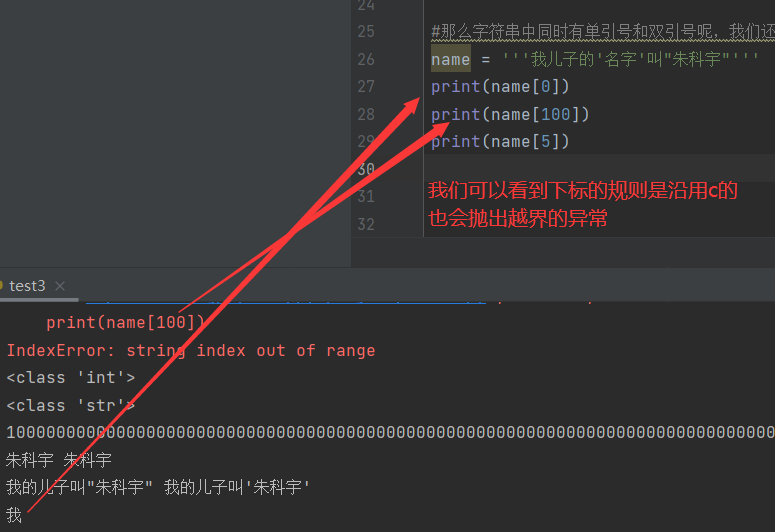``````#那么字符串中同时有单引号和双引号呢，我们还可以用三引号括起来
name = '''我儿子的'名字'叫"朱科宇"'''
print(name)
print(name)
print(name)
``````

与c极大不同的地方 下标可以为负数

下标为-1就等价于下标为len-1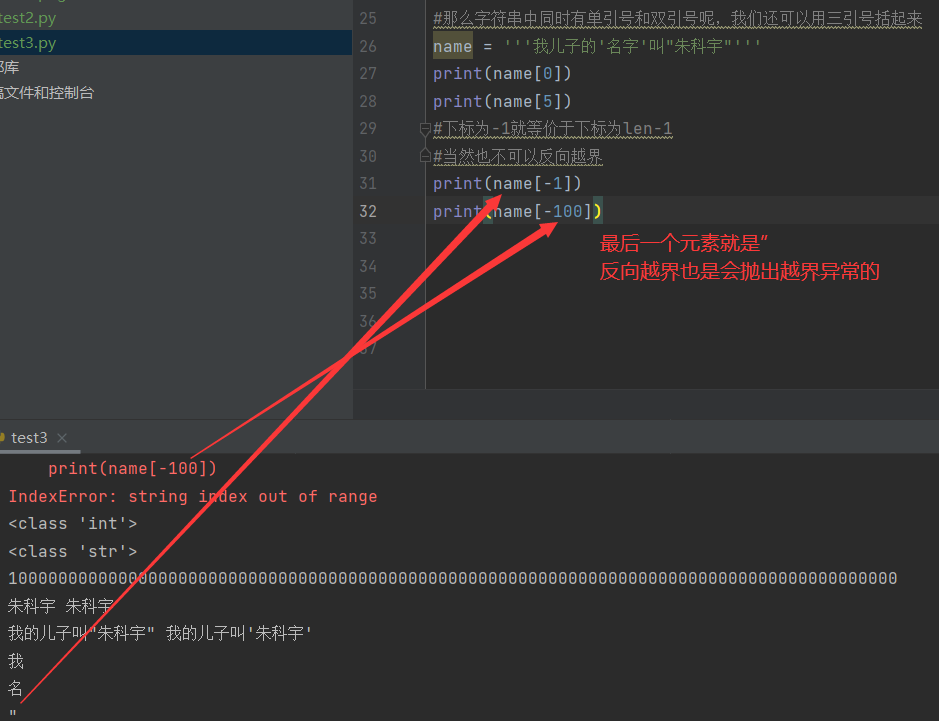``````#下标为-1就等价于下标为len-1
#当然也不可以反向越界
print(name[-1])
print(name[-100])
``````

切片操作符 [:]来获取子字符串(切片操作是一个前闭后开区间).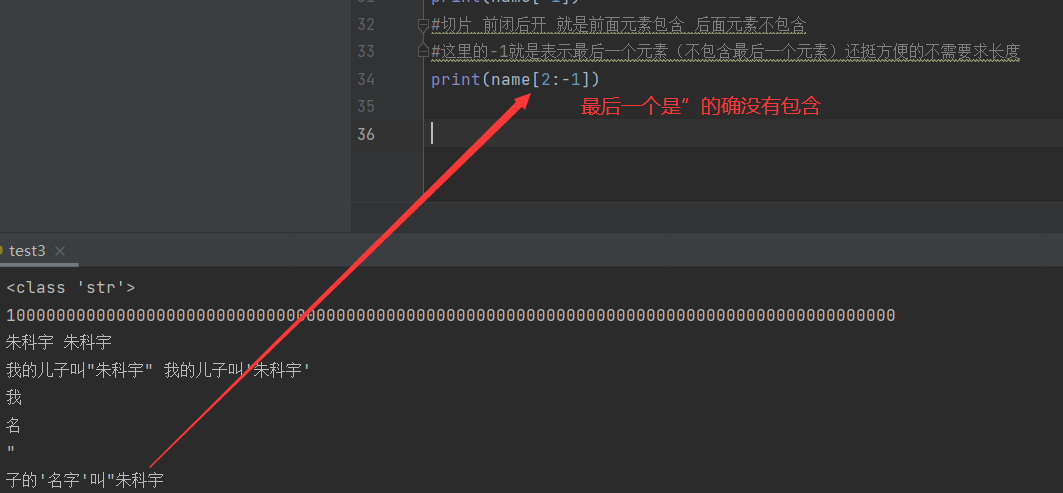``````#切片 前闭后开 就是前面元素包含 后面元素不包含
#这里的-1就是表示最后一个元素（不包含最后一个元素）还挺方便的不需要求长度
print(name[2:-1])
``````

当然也可以省略

1. 省略前面就是从开始的地方算
2. 省略后面就是一直到最后面
3. 都省略就是和原串一样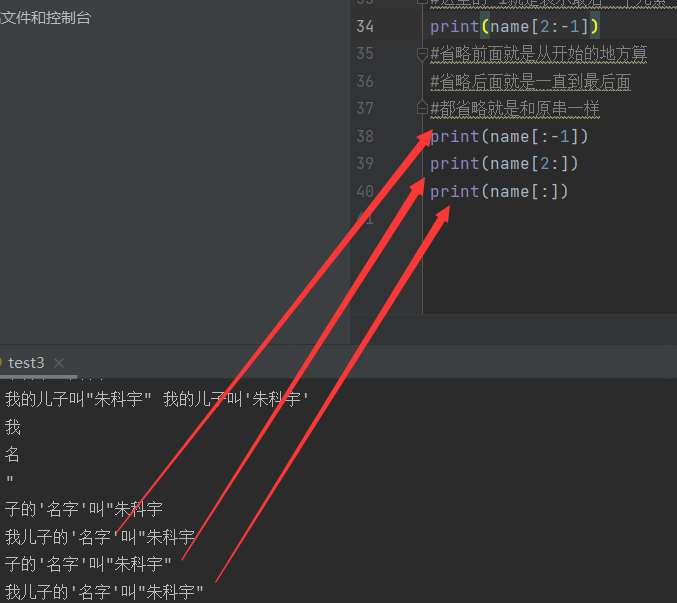``````#省略前面就是从开始的地方算
#省略后面就是一直到最后面
#都省略就是和原串一样
print(name[:-1])
print(name[2:])
print(name[:])
``````

==+==用于字符串拼接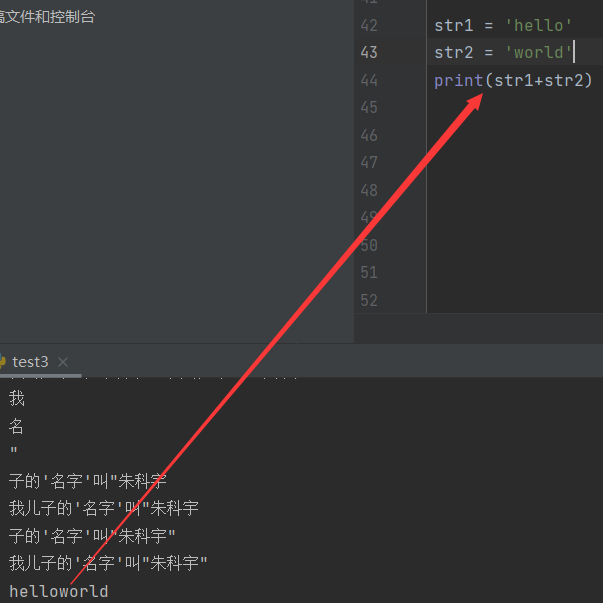``````str1 = 'hello'
str2 = 'world'
print(str1+str2)
``````

==*==用于字符串重复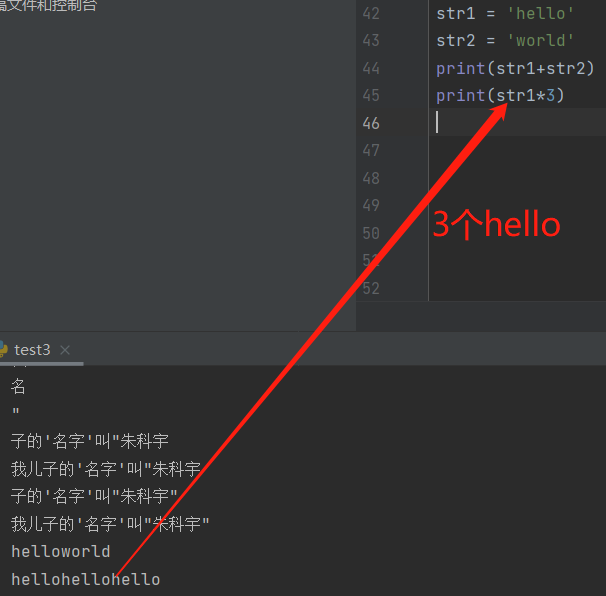``````str1 = 'hello'
str2 = 'world'
print(str1+str2)
print(str1*3)
``````

## 认识 “布尔类型”

Python中用TrueFalse来表示布尔值(注意, 第一个字符大写).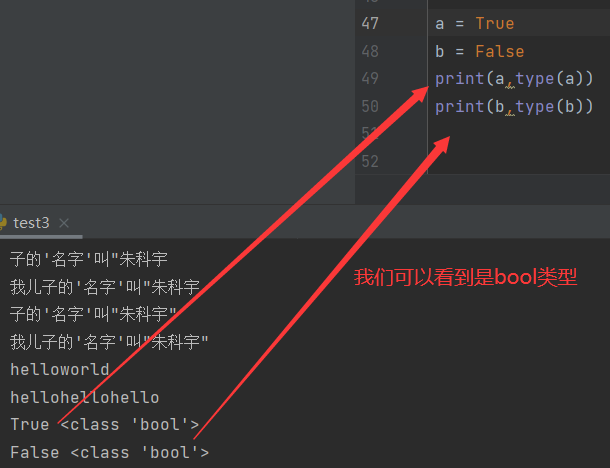``````a = True
b = False
print(a,type(a))
print(b,type(b))
``````

布尔类型的变量, 也是一种特殊的整数类型. 在和整数进行运算时, True被当做1, False被当做0.和c一样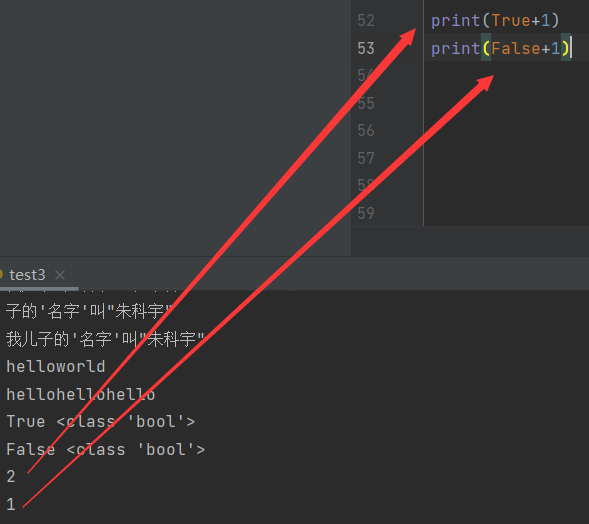``````print(True+1)
print(False+1)
``````

来源：小码农UU